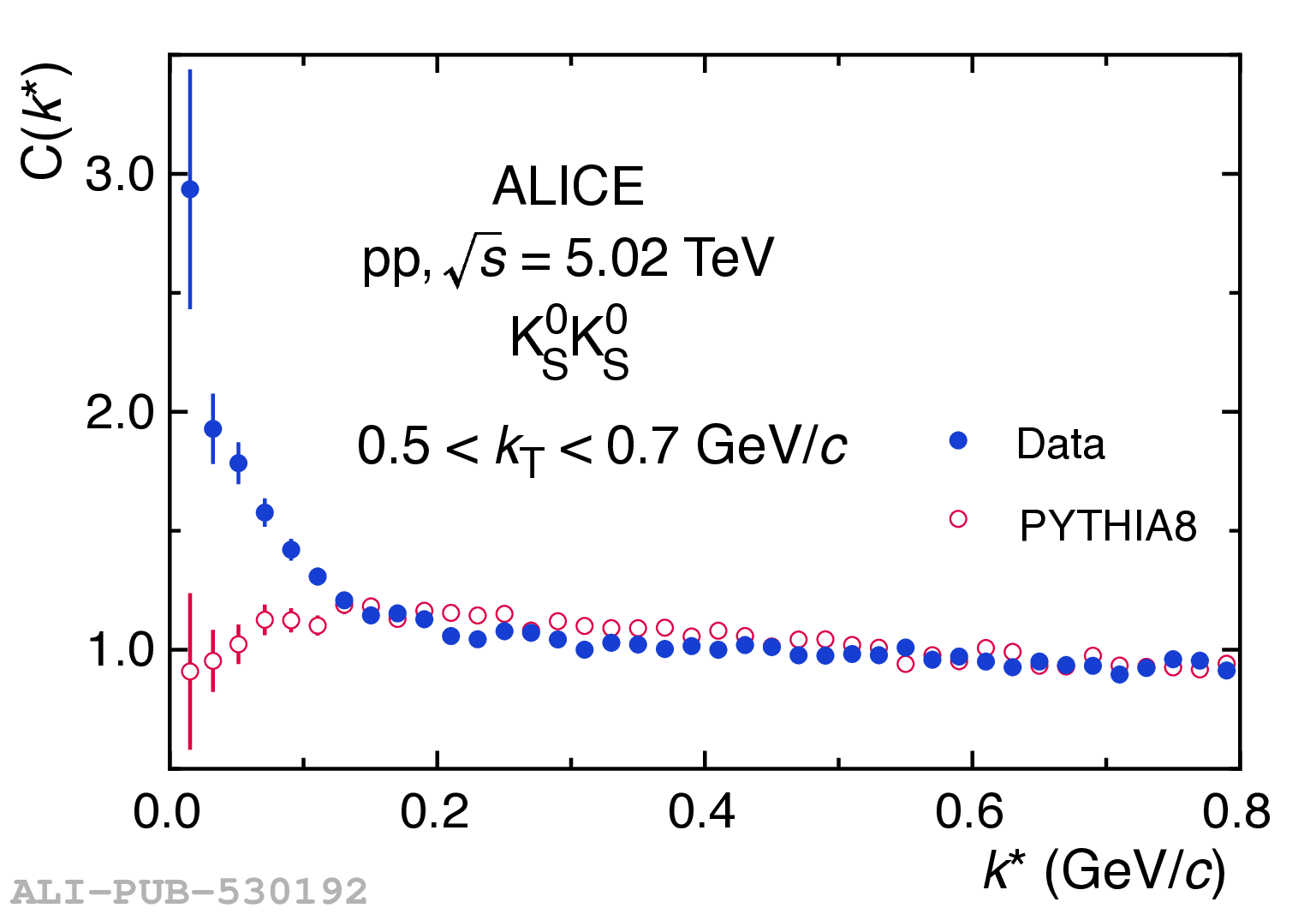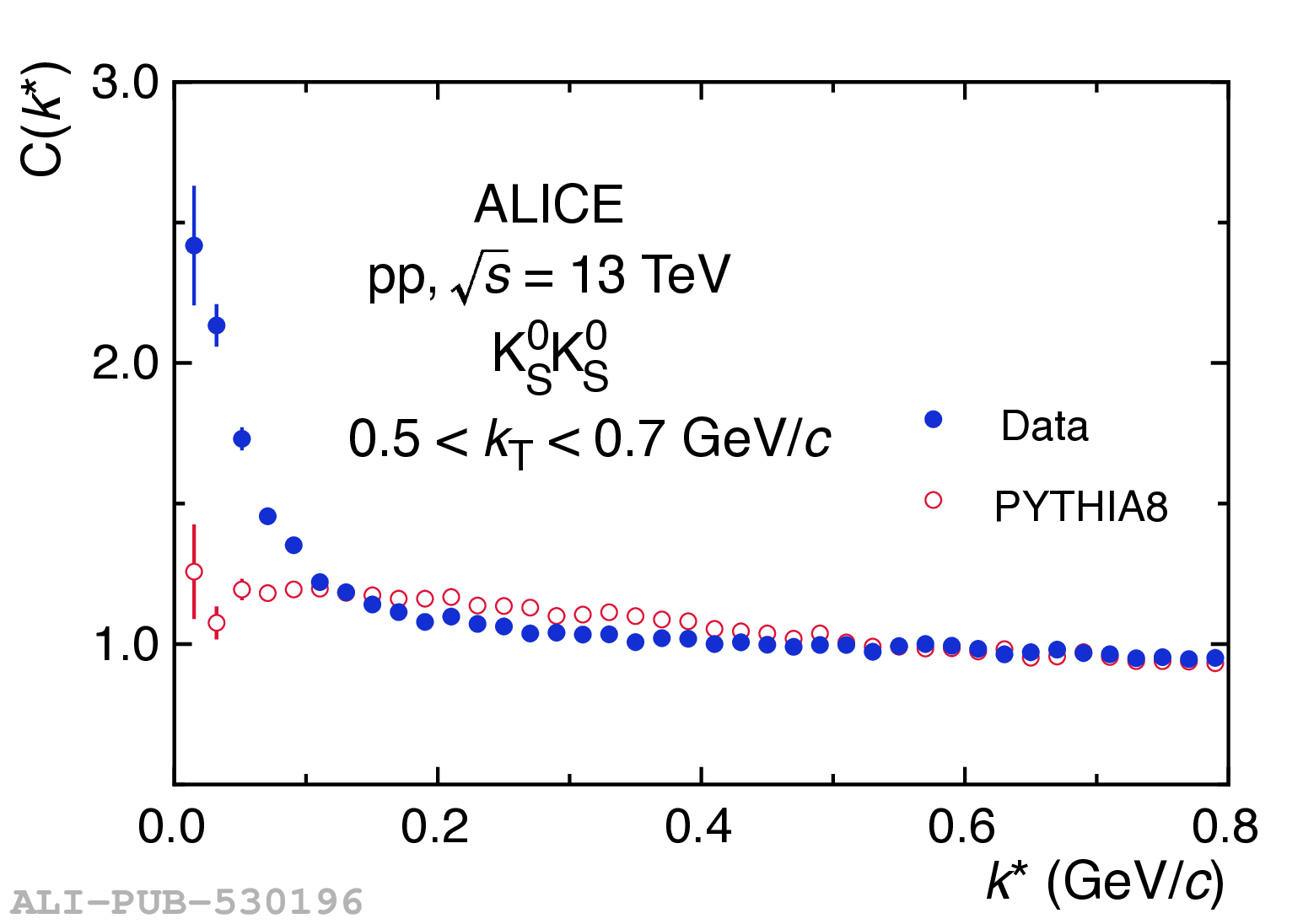# Figure 1

 Example K$^0_{\rm S}$K$^0_{\rm S}$ correlation functions along with the resulting distributions from PYTHIA8 simulationsin pp collisions at $\sqrt{s}=5.02$ (left) and $13$ TeV (right).